Courses

# Micro Cracking of Concrete, Behavior under Multiaxial Loading Civil Engineering (CE) Notes | EduRev

## Civil Engineering (CE) : Micro Cracking of Concrete, Behavior under Multiaxial Loading Civil Engineering (CE) Notes | EduRev

The document Micro Cracking of Concrete, Behavior under Multiaxial Loading Civil Engineering (CE) Notes | EduRev is a part of Civil Engineering (CE) category.
All you need of Civil Engineering (CE) at this link: Civil Engineering (CE)

Micro-cracking of Concrete / Behavior under Multiaxial Loading
→ Strength properties of concrete
o Concrete is a complex material consisting of coarse aggregate, sand, cement gel, unhydrated cement particles, capillary and gel pores, pore water, air voids etc.
o Concrete is multi-phased at several levels:

• Micro-level: multi-element
• Meso-level: multi-phase
• Macro-level: homogeneous

o A mortar-aggregate system is considered to be at meso-level.
o Cracking stages:
1. Bond cracks at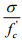≅ 0.3
2. Mortar cracks at≅ 0.75 0.80.
3. Unstable crack propagation
4. Failure

where  fc' = reference strength, which is usually obtained from uniaxial testing (compression) of standard size cylinders.

→  Stress-strain behavior of concrete
o Stress-strain (σ −ε ) relation is used to determine material property, such as the modulus of elasticity (Young’s modulus), E, strength, and failure strain as a basis for analysis and design purposes.
o For concrete, since it is mainly used in compression, the modulus of elasticity, Ec , is determined from compressive stress-strain curves that are generally obtained from uniaxial compressive tests.

→  Deflection and failure behavior as affected by microcracking
o Model study:
Deformation and fracture of a concrete model: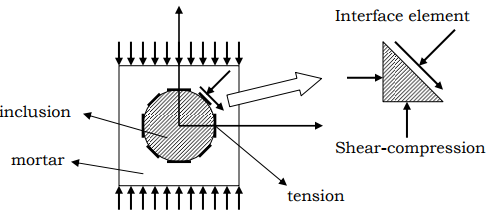o Interface elements are used to study the influence of matrix-inclusion that is bond effect and particle-interaction phenomena in modeling.
o Precise analysis should account for the influence of nonhomogeneity, models of interfacial behavior, and include recognition of interface debonding and resulting progressive fracture.
o Sophisticated analysis shows that bond failure initiates due to compression-shear and that the failure behavior is controlled by microcracking due to direct tensile stresses and splitting of concrete.

o Two debonding modes:
1. Compression-shear (including pure shear) failure at interface
2. Tensile-shear (including pure tension) debonding

Compression-shear strength
o The compression-shear strength of concrete is based on:
1. Chemical bond which is an attraction between the mortar and aggregate constituents.
2. Mechanical bond which results from the interlocking of the mortar and the aggregate in the irregularities of the aggregate surfaces.

→ Uniaxial compression
o Inelastic volume increase → “dilatancy”
o The behavior is related to microcracking.

→ Uniaxial tension
o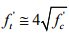→ Bending test
o Bending test
Modulus of rupture: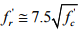→ Strain rate effects

→ Stress-strain under cyclic loading

→ Behavior of concrete in combined stress
o In many structural situations concrete is subjected to multiaxial state of stress.
Beams: tension, compression + shear
Slabs: compression + shear
Thin shells: biaxial stresses
Thick shells (containments etc.): triaxial stresses

→ Any combined stress state can be reduced to three normal stresses acting on three mutually perpendicular planes. These three normal stresses are the principal stresses, and the shear stresses acting on these planes are zero (eigenvalue problem). Thus, uniaxial, biaxial, and triaxial stress states are considered.

o Uniaxial loading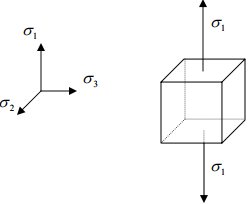σ1 , σ2 , σ3 are principal stresses.
σ2 , σ3 = 0 → unaxial condition,
σ1 < 0 → uniaxial compression,
σ1 > 0 → uniaxial tension.

o  The strength of concrete depends on the type of mixture, the properties of aggregate, and the time and quality of curing. Concrete is neither homogeneous nor isotropic. The non-isotropic feature of concrete makes its compressive strength different from its tensile strength.

o  Uniaxial compressive strength
The compressive strength, f,c, is obtained on standard 6 in.-by-12 in. cylinders cured under standard laboratory conditions and tested at a specified loading rate at 28 days of age.

Normal strength concrete is considered to have a compressive strength range from 3000 psi to 6000 psi. (Discussion in this outline pertains to the behavior of normal strength concrete.)

ACI Code suggests: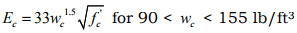where w= the density of concrete. For normal weight concrete,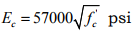“Dilatancy”: Inelastic volume increase.
→ It is related to microcracking and brings a complex deformation and failure behavior including dependency on hydrostatic pressure in failure.
→ Uniaxial tensile strength Cylinder splitting test – Tensile splitting strength, 'ft .
→ Flexural test
ACI Code suggests, for normal weight concrete,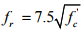(modulus of rupture)

and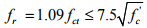for lightweight concrete, where fct = splitting tensile strength.

→ Short-time response in compressive loading tests
From the uniaxially compressive testing results, the short-time response curves consist of an initial relatively straight-line portion and a curve-to-level portion that contains the maximum stress. The curve descends after the maximum stress is reached. Different ways of testing result in different characteristics of the after-peak behavior.
Maximum strain for normal density concrete generally ranges from 0.002 to 0.003 and from 0.003 to 0.0035 for lightweight concrete. In general, the modulus of elasticity calculated from the initial straight portion of the stress-strain curve is larger when the strength of the concrete is higher.

→Testing conditions, such as specimen boundary condition and loading rate, influence stress-strain curve characteristics.

→ Long-time response in compressive loading tests
(a) The influence of creep deformation → concrete deforms under sustained load.
(b) The influence of fatigue → the fatigue strength of concrete depends not only on the static strength of concrete but also on moisture condition, age, and rate and range of loading.

→ Several observations on the stress-strain curves of concrete of various strengths by the Portland Cement Association (PCA):
(a) The lower the strength of concrete, the higher the failure strain.
(b) The length of the initial relatively linear portion increases with the increase in the compressive strength of concrete.
(c) There is a marked reduction in ductility with increased strength.

o Biaxial stress state
→ Effect of microcracking ƒ Biaxial stress state is considered with principal stresses acting only in two directions.
→ When concrete is compressed in biaxial stress state:
1. The observed compressive strength increases.
2. The tensile ductility is greater than that under unaxial compression. For compression-tension region ductility decreases with the increase in tension.
3. Elastic limit is shifted up.
4. As the failure point is approached, an increase in volume occurs as the compressive stress continues to increase. In biaxial compression volumetric strains increase.
5. Failure modes depend on various stress combinations.

o Biaxial loading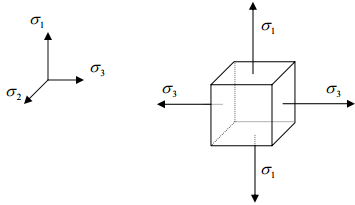o  σ2 = 0 → biaxial condition,
σ1 < 0 and σ3 < 0 → biaxial compression,
σ1 > 0 and σ3 > 0 → biaxial tension,
σ1 < 0 and σ3 > 0 or σ 1 > 0 and σ3 < 0 → shear.

→ Various forms of analytical expression of the strength of concrete under combined stress (biaxial stress) have been proposed.
→ The biaxial strength of concrete in compression is higher than its uniaxial strength.
→ The biaxial strength of concrete in tension is lower than its uniaxial tension strength.

o Constitutive equation
→ The constitutive equation for an isotropic elastic material under biaxial stresses: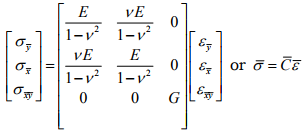where σy , σx , σxy = stresses in the local coordinate systems,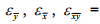strains in the local coordinate systems,
E = modulus of elasticity,
G = shear modulus,
ν = Poisson’s ratio, and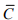= the biaxial constitutive matrix of an isotropic, continuous, and elastic material.

→ The constitutive matrix accounting for the compression-shear cracks
(a) In the local coordinate systems: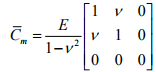→ Note that actually the shear modulus is a non-zero value, αG , to reflect the effect of shear transfer across cracked surfaces (aggregate interlock, dowel action etc.) (α <1). See outline on shear transfer.
(b) In the global coordinate systems: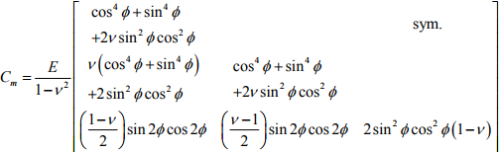→ The constitutive matrix accounting for the tensile-shear cracks in the local coordinate systems:
(a) In the local coordinate systems: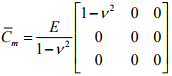(b) In the global coordinate systems: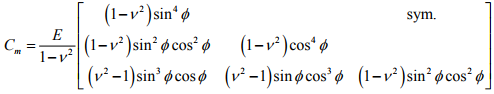→ Deformational and fractural behavior of the analytical model under uniaxial compressive stress is affected by debonding at the interface between the matrix and the inclusions.
→ Fracture occurs by progressive cracking, starting at the interface and resulting in separation between the two constituents.
→ Ultimate failure is obtained by progression of the interfacial cracks into the matrix, forming crack patterns parallel to the loading direction.
→ Bond failure at the interface, resulting in local deformation accumulation, affects the overall nonlinear deformational behavior.

o Triaxial loading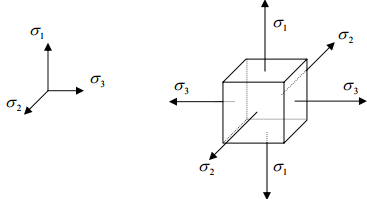→ In normal strength concrete fracture occurs by progressive cracking, starting at the interface and resulting in separation between the two constituents.

σ1 , σ2 , σ3 ≠ 0 → triaxial condition,
σ1 < 0 and σ2 < 0 and σ3 < 0 → triaxial compression,
σ1 > 0 and σ2 > 0 and σ3 > 0 → triaxial tension,
1 < 0 and σ2 > 0 and σ3 > 0} or {σ1 > 0 and σ2 < 0 and σ3 < 0} or
1 > 0 and σ2 < 0 and σ3 > 0} or {σ1 < 0 and σ2 > 0 and σ3 < 0} or
1 > 0 and σ2 > 0 and σ3 < 0} or {σ1 < 0 and σ2 < 0 and σ3 > 0}
→ triaxial shear.

o  Nilson (1997) reported several observations regarding the triaxial strength of concrete:
“1. In a state of equal triaxial compression, concrete strength may be an order of magnitude larger than the uniaxial compressive strength.
2. For equal biaxial compression combined with a smaller value of compression in the third direction, a strength increase greater than 20 percent can be expected.
3. For stress states including compression combined with tension in at least one other direction, the intermediate principal stress is of little consequence, and the compressive strength can be predicted safely…”

o  Failure of concrete in triaxial stress state
1. The failure surface in triaxial stress state is described by a hydro-axis and deviatoric sections. Intersection of the failure surface with σ3 = 0 plane gives σ1 - σ2 biaxial failure curve. Other representations involve failure description in deviatoric and hydrostatic planes.
2. Under high confining stress the possibility of bond cracking is reduced; and failure mode shifts from cleavage to crushing of the cement paste.
3. Concrete is a brittle material which fails through brittle cleavage (splitting) at the interfaces and in mortar except for high triaxial compression where shear slippage occurs resulting in a ductile behavior.

o The strength of concrete under combined stress cannot be determined analytically, in a precise manner, mainly due to the following reasons:
1. The existence of cracks in concrete makes it become nonhomogeneous and non-isotropic. Traditional approach using continuum mechanics cannot be directly applied to develop the analytical expression of strength of concrete in this circumstance. Currently the prediction of concrete strength under combined stress relies on experimental results.
2. In many situations it is not possible to determine all the acting stresses and their directions in concrete structures.

→  Concrete confinement by transverse and longitudinal reinforcement
o Different confinement scenarios that may be used in practice are illustrated below: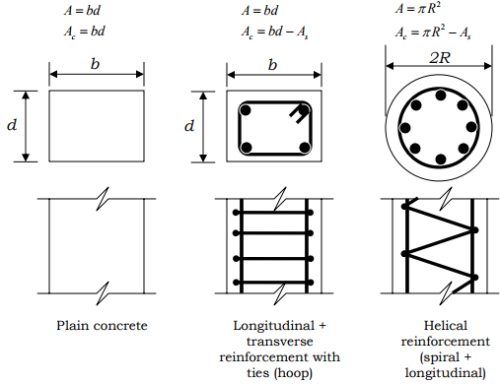→ Smaller spacings result in more effective confinement.
→ Closely spaced spirals may result near uniform pressure.
→ Tests have shown that transverse reinforcement can considerably improve the stress-strain characteristics at high strains.

o In practice, concrete may be confined by transverse reinforcement (in combination with the longitudinal reinforcement); Closely spaced steel spirals or hoops.
o Concrete becomes confined when at stresses approaching the uniaxial strength, the transverse strains become very high and concrete bears out against the transverse reinforcement.

o Effect of different confinement scenarios on axial capacity
Total axial capacity: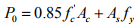→ The factor 0.85 is used for vertically cast members for the effects of:
–– bleeding in long columns (strength variation along the axis)
–– eccentricity effects
–– size effects

→ The effect of the spiral is comparable to the specimens subjected to lateral pressure f2 in which case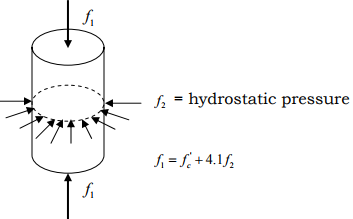e.g. for f2 = 1000 psi, f'c = 3000 psi, f1 ≅ 7000 psi.
If h = core diameter (out-to-out) and An = core area, the above result implies that the contribution of the lateral pressure to the ultimate load = 4.1 f2 An .
→ Define Ab = area of spiral and s = pitch, then the volumetric steel percentage is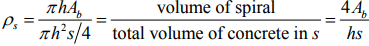→ The lateral confining pressure may be expressed approximately in terms of the tension in spiral reinforcement.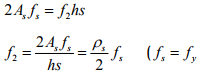in tension at failure)
Since the contribution of the lateral pressure to the ultimate axial load = 4.1 f2 An .it turns out that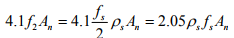Validity of this equation has been verified by the tests where the coefficient ranged from 1.7 to 2.9.

→ When P0 is reached, the shell of concrete outside the core will fail and the load capacity of the column will reduce. But due to confinement no buckling will occur in the longitudinal steel.

→ ACI recommends that the contribution of the transverse reinforcement to the strength should be at least equal to the contribution of the concrete cover.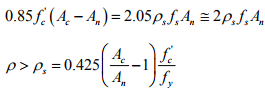→ Other confinement mechanism
o Confinement by steel shells and FRP sheets. Discuss various models.

→ Failure theories for concrete under multiaxial loading and constitutive relations are summarized in Outline 3.

Offer running on EduRev: Apply code STAYHOME200 to get INR 200 off on our premium plan EduRev Infinity!

,

,

,

,

,

,

,

,

,

,

,

,

,

,

,

,

,

,

,

,

,

,

,

,

;